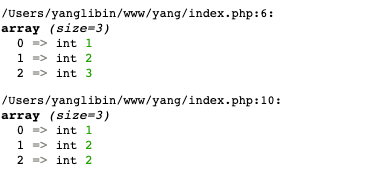PHP中foreach用&出现的问题

$arr = [1, 2, 3]; foreach ($arr as $key => &$v) {
$a = 1; } var_dump($arr);
foreach ($arr as$key => $v) {$a = 1;
}
var_dump($arr);跟预期的结果不太一样，查了下资料，原因就在于foreach中的引用在foreach完成后并没有释放，也就是说在第一个foreach中的引用一直保留着，当第一个foreach完成后，$v是指向$arr的一个引用，修改$v会修改$arr的值，再第二个foreach中，不断的将$arr中的值赋给$v，即第二个foreach执行中$arr的值在不断地变化，首先是$arr赋给$v，$v修改$arr的值，变为1，然后是$arr赋给$v,$v修改$arr的值，变为2。

$arr = [1,2,3]; foreach($arr as &$value) {//执行完这段代码后$value是指向$arr的一个引用，修改$value会修改$arr的值 } foreach($arr as $value) { print_r($arr);
}

Array

(
 => 1
 => 2
 => 1
)
Array
(
 => 1
 => 2
 => 2
)
Array
(
 => 1
 => 2
 => 2
)

foreach($arr as &$value) {//执行完这段代码后 $value是指向$arr的一个引用，修改$value会修改$arr的值

}

posted on 2020-06-18 12:00  Ryanyanglibin  阅读(235)  评论(0编辑  收藏  举报